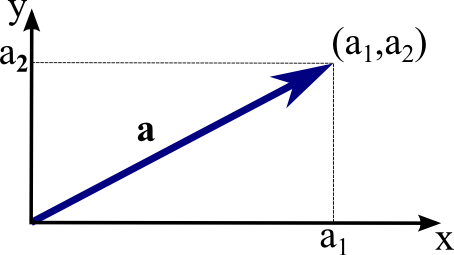# Math Insight

### Image: The coordinates of a vector in two dimensionsTo determine the coordinates of a two-dimensional vector $\vc{a}$, translate its tail to the origin. The head of $\vc{a}$ will be at some point $(a_1,a_2)$ in the Cartesian coordinate system. We call $(a_1,a_2)$ the coordinates or the components of the vector $\vc{a}$.

Image file: vector_2d_coordinates.png

Source image file: vector_2d_coordinates.svg
Source image type: Inkscape SVG